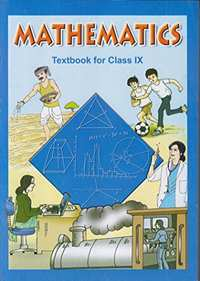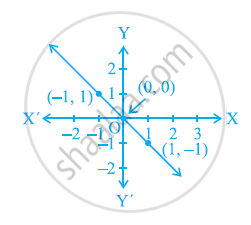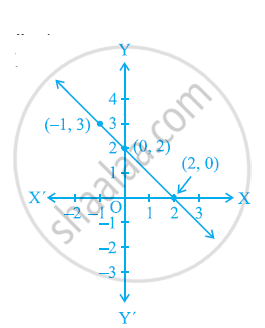# NCERT solutions for Class 9 Maths chapter 4 - Linear Equations in two Variables [Latest edition]

#### Chapters## Solutions for Chapter 4: Linear Equations in two Variables

Below listed, you can find solutions for Chapter 4 of CBSE NCERT for Class 9 Maths.

Exercise 4.1Exercise 4.2Exercise 4.3Exercise 4.4
Exercise 4.1 [Page 68]

### NCERT solutions for Class 9 Maths Chapter 4 Linear Equations in two Variables Exercise 4.1 [Page 68]

Exercise 4.1 | Q 1 | Page 68

The cost of a notebook is twice the cost of a pen. Write a linear equation in two variables to represent this statement.

(Take the cost of a notebook to be Rs. x and that of a pen to be Rs. y).

Exercise 4.1 | Q 2.1 | Page 68

Express the following linear equation in the form ax + by + c = 0 and indicate the values of a, b and c in case:- 2x+3y=9.3bar5

Exercise 4.1 | Q 2.2 | Page 68

Express the following linear equation in the form ax + by + c = 0 and indicate the values of a, b and c in case:- x-y/5-10=0

Exercise 4.1 | Q 2.3 | Page 68

Express the following linear equation in the form ax + by + c = 0 and indicate the values of a, b and c in case:- –2x + 3y = 6

Exercise 4.1 | Q 2.4 | Page 68

Express the following linear equation in the form ax + by + c = 0 and indicate the values of a, b and c in case:- x = 3y

Exercise 4.1 | Q 2.5 | Page 68

Express the following linear equation in the form ax + by + c = 0 and indicate the values of a, b and c in case:-

2x = –5y

Exercise 4.1 | Q 2.6 | Page 68

Express the following linear equation in the form ax + by + c = 0 and indicate the values of a, b and c in case:-

3x + 2 = 0

Exercise 4.1 | Q 2.7 | Page 68

Express the following linear equation in the form ax + by + c = 0 and indicate the values of a, b and c in case:-

y – 2 = 0

Exercise 4.1 | Q 2.8 | Page 68

Express the following linear equation in the form ax + by + c = 0 and indicate the values of a, b and c in case:-

5 = 2x

Exercise 4.2 [Page 70]

### NCERT solutions for Class 9 Maths Chapter 4 Linear Equations in two Variables Exercise 4.2 [Page 70]

Exercise 4.2 | Q 1 | Page 70

Which one of the following options is true, and why?

y = 3x + 5 has

(i) a unique solution, (ii) only two solutions, (iii) infinitely many solutions

Exercise 4.2 | Q 2.1 | Page 70

Write four solutions for the following equation:- 2x + y = 7

Exercise 4.2 | Q 2.2 | Page 70

Write four solutions for the following equation:- πx + y = 9

Exercise 4.2 | Q 2.3 | Page 70

Write four solutions for the following equation:- x = 4y

Exercise 4.2 | Q 3.1 | Page 70

Check if (0, 2) is the solution of the equation x - 2y = 4.

Exercise 4.2 | Q 3.2 | Page 70

Check if (2, 0) is the solution of the equation x - 2y = 4.

Exercise 4.2 | Q 3.3 | Page 70

Check if (4, 0) is the solution of the equation x - 2y = 4.

Exercise 4.2 | Q 3.4 | Page 70

Check if (sqrt2", "4sqrt2) is the solution of the equation x - 2y = 4.

Exercise 4.2 | Q 3.5 | Page 70

Check if (1, 1) is the solution of the equation x - 2y = 4.

Exercise 4.2 | Q 4 | Page 70

Find the value of k, if x = 2, y = 1 is a solution of the equation 2x + 3y = k.

Exercise 4.3 [Page 74]

### NCERT solutions for Class 9 Maths Chapter 4 Linear Equations in two Variables Exercise 4.3 [Page 74]

Exercise 4.3 | Q 1.1 | Page 74

Draw the graph of the following linear equations in two variables:- x + y = 4

Exercise 4.3 | Q 1.2 | Page 74

Draw the graph of the following linear equations in two variables:- x – y = 2

Exercise 4.3 | Q 1.3 | Page 74

Draw the graph of the following linear equations in two variables:- y = 3x

Exercise 4.3 | Q 1.4 | Page 74

Draw the graph of the following linear equations in two variables:- 3 = 2x + y

Exercise 4.3 | Q 2 | Page 74

Give the equations of two lines passing through (2, 14). How many more such lines are there, and why?

Exercise 4.3 | Q 3 | Page 74

If the point (3, 4) lies on the graph of the equation 3y = ax + 7, find the value of a.

Exercise 4.3 | Q 4 | Page 74

The taxi fare in a city is as follows:- For the first kilometre, the fare is Rs. 8 and for the subsequent distance it is Rs. 5 per km. Taking the distance covered as x km and total fare as Rs y, write a linear equation for this information, and draw its graph.

Exercise 4.3 | Q 5 | Page 74

From the choices given below, choose the equation whose graphs are given in the given figures.

For the first figure

(i) y = x

(ii) x y = 0

(iii) y = 2x

(iv) 2 + 3y = 7xFor the second figure

(i) y = x +2

(ii) y = x − 2

(iii) y = − x + 2

(iv) x + 2y = 6Exercise 4.3 | Q 6 | Page 74

If the work done by a body on application of a constant force is directly proportional to the distance travelled by the body, express this in the form of an equation in two variables and draw the graph of the same by taking the constant force as 5 units. Also read from the graph the work done when the distance travelled by the body is

(i) 2 units (ii) 0 unit

Exercise 4.3 | Q 7 | Page 74

Yamini and Fatima, two students of Class IX of a school, together contributed Rs. 100 towards the Prime Minister’s Relief Fund to help the earthquake victims. Write a linear equation which satisfies this data. (You may take their contributions as Rs. x and Rs. y.) Draw the graph of the same.

Exercise 4.3 | Q 8 | Page 74

In countries like USA and Canada, temperature is measured in Fahrenheit, whereas in countries like India, it is measured in Celsius. Here is a linear equation that converts Fahrenheit to Celsius:-

F=(9/5)C+32

(i) Draw the graph of the linear equation above using Celsius for x-axis and Fahrenheit for y-axis.

(ii) If the temperature is 30°C, what is the temperature in Fahrenheit?

(iii) If the temperature is 95°F, what is the temperature in Celsius?

(iv) If the temperature is 0°C, what is the temperature in Fahrenheit and if the temperature is 0°F, what is the temperature in Celsius?

(v) Is there a temperature which is numerically the same in both Fahrenheit and Celsius? If yes, find it.

Exercise 4.4 [Page 77]

### NCERT solutions for Class 9 Maths Chapter 4 Linear Equations in two Variables Exercise 4.4 [Page 77]

Exercise 4.4 | Q 1.1 | Page 77

Give the geometric representations of y = 3 as an equation in one variable.

Exercise 4.4 | Q 1.2 | Page 77

Give the geometric representations of y = 3 as an equation in two variables.

Exercise 4.4 | Q 2.1 | Page 77

Give the geometric representations of 2x + 9 = 0 as an equation in one variable.

Exercise 4.4 | Q 2.2 | Page 77

Give the geometric representations of 2x + 9 = 0 as an equation in two variables.

## Solutions for Chapter 4: Linear Equations in two Variables

Exercise 4.1Exercise 4.2Exercise 4.3Exercise 4.4## NCERT solutions for Class 9 Maths chapter 4 - Linear Equations in two Variables

Shaalaa.com has the CBSE Mathematics Class 9 Maths CBSE solutions in a manner that help students grasp basic concepts better and faster. The detailed, step-by-step solutions will help you understand the concepts better and clarify any confusion. NCERT solutions for Mathematics Class 9 Maths CBSE 4 (Linear Equations in two Variables) include all questions with answers and detailed explanations. This will clear students' doubts about questions and improve their application skills while preparing for board exams.

Further, we at Shaalaa.com provide such solutions so students can prepare for written exams. NCERT textbook solutions can be a core help for self-study and provide excellent self-help guidance for students.

Concepts covered in Class 9 Maths chapter 4 Linear Equations in two Variables are Solution of a Linear Equation, Graph of a Linear Equation in Two Variables, Linear Equation in Two Variables, Equations of Lines Parallel to the X-axis and Y-axis, Linear Equation in One Variable.

Using NCERT Class 9 Maths solutions Linear Equations in two Variables exercise by students is an easy way to prepare for the exams, as they involve solutions arranged chapter-wise and also page-wise. The questions involved in NCERT Solutions are essential questions that can be asked in the final exam. Maximum CBSE Class 9 Maths students prefer NCERT Textbook Solutions to score more in exams.

Get the free view of Chapter 4, Linear Equations in two Variables Class 9 Maths additional questions for Mathematics Class 9 Maths CBSE, and you can use Shaalaa.com to keep it handy for your exam preparation.

Share